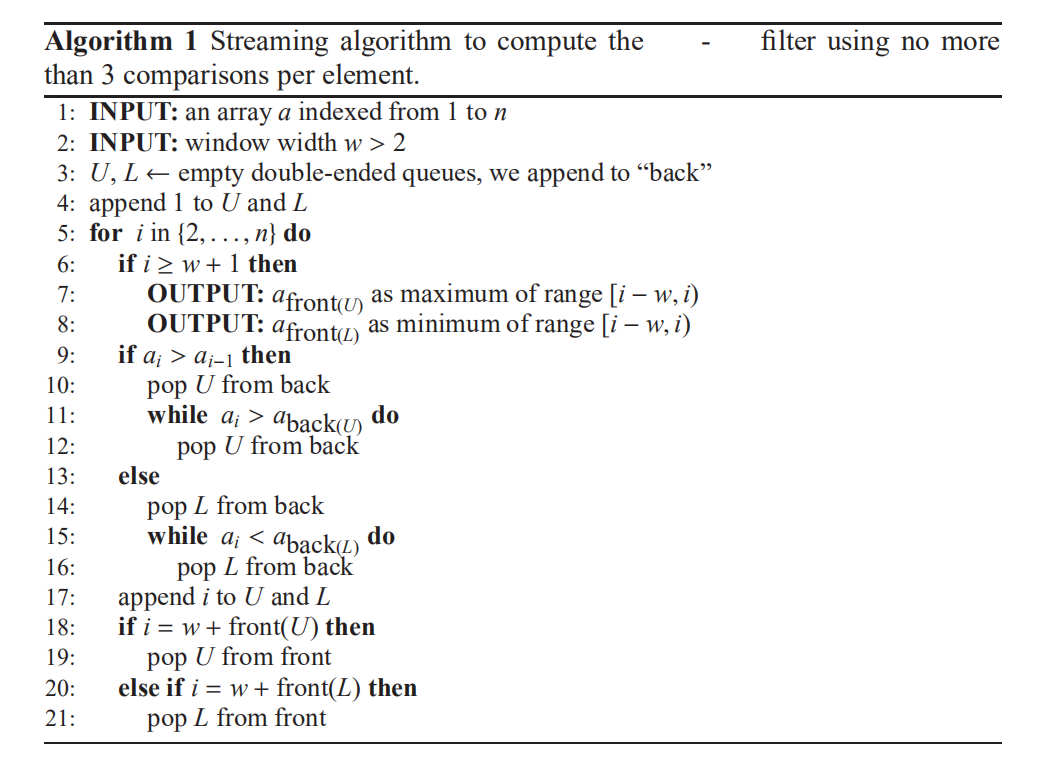# 3. 算法原理¶其实算法也是比较好理解的，即动态维护一个长度为$r$（滤波窗口大小）的单调队列，然后可以在任意位置获取以当前点为结束点的滤波窗口中的最大值或者最小值。

# 4. 代码实现¶

#include <bits/stdc++.h>
using namespace std;
const int maxn = 110;
deque <int> U, L;
int maxval[maxn], minval[maxn];

int main(){
int a = {0, 1, 9, 8, 2, 3, 7, 6, 4, 5};
int w = 3;
for(int i = 1; i < 10; i++){
if(i >= w){
maxval[i - w] = a[U.size() > 0 ? U.front() : i-1];
minval[i - w] = a[L.size() > 0 ? L.front() : i-1];
}
if(a[i] > a[i-1]){
L.push_back(i - 1);
if(i == w + L.front()) L.pop_front();
while(U.size() > 0){
if(a[i] <= a[U.back()]){
if(i == w + U.front()) U.pop_front();
break;
}
U.pop_back();
}
}
else{
U.push_back(i-1);
if(i == w + U.front()) U.pop_front();
while(L.size() > 0){
if(a[i] >= a[L.back()]){
if(i == w + L.front()) L.pop_front();
break;
}
L.pop_back();
}
}
}
maxval[10 - w] = a[U.size() > 0 ? U.front() : 9];
minval[10 - w] = a[L.size() > 0 ? L.front() : 9];
for(int i = 0; i <= 10 - w; i++){
printf("Min index %d is: %d\n", i, minval[i]);
printf("Max index %d is: %d\n", i, maxval[i]);
}
return 0;
}


# 6. 总结¶